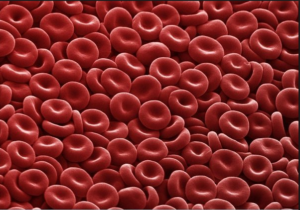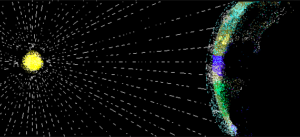## 0.3 Scientific Notation

### QUICK REFERENCE

• Scientific Notation is of the general form $a\times10^{n}$, where $1\leq{a}<10$ and n is an integer.
• To multiply numbers in scientific notation multiply the numbers that aren’t powers of 10 (the a in $a\times10^{n}$). Then multiply the powers of ten by adding the exponents.
• To divide numbers in scientific notation divide the numbers that aren’t powers of 10 (the a in $a\times10^{n}$. Then divide the powers of ten by subtracting the exponents.
• Comparing numbers written in scientific notation:

$5\times10^{2}$ is smaller than $4.3\times10^{7}$

With positive exponents, the larger the exponent, the larger the number.

$5\times10^{-2}$ is larger than $4.3\times10^{-7}$

With negative exponents, the larger the negative exponent, the smaller the number.

## Convert between scientific and decimal notation

Scientific notation is used by scientists, mathematicians, and engineers when they are working with very large or very small numbers. Using exponential notation, large and small numbers can be written in a way that is easier to read.

When a number is written in scientific notation, the exponent tells you if the term is a large or a small number. A positive exponent indicates a large number and a negative exponent indicates a small number between 0 and 1.

A light year is the number of miles light travels in one year, about 5,880,000,000,000.  That’s a lot of zeros, and it is easy to lose count when trying to figure out the place value of the number. Using scientific notation, the distance is $5.88\times10^{12}$ miles. The exponent of 12 tells us how many places to count to the left of the decimal. Another example of how scientific notation can make numbers easier to read is the diameter of a hydrogen atom, which is about 0.00000005 mm, and in scientific notation is $5\times10^{-8}$ mm. In this case the $-8$ tells us how many places to count to the right of the decimal.

### Scientific Notation

A positive number is written in scientific notation if it is written as $a\times10^{n}$ where the coefficient a is $1\leq{a}<10$, and n is an integer.

Now let’s compare some numbers expressed in both scientific notation and standard decimal notation in order to understand how to convert from one form to the other. Take a look at the tables below. Pay close attention to the exponent in the scientific notation and the position of the decimal point in the decimal notation.

 Large Numbers Small Numbers Decimal Notation Scientific Notation Decimal Notation Scientific Notation 500.0 $5\times10^{2}$ 0.05 $5\times10^{-2}$ 80,000.0 $8\times10^{4}$ 0.0008 $8\times10^{-4}$ 43,000,000.0 $4.3\times10^{7}$ 0.00000043 $4.3\times10^{-7}$ 62,500,000,000.0 $6.25\times10^{10}$ 0.000000000625 $6.25\times10^{-10}$

### Convert from decimal notation to scientific notation

To write a large number in scientific notation, move the decimal point to the left to obtain a number between 1 and 10. Since moving the decimal point changes the value, you have to multiply the decimal by a power of 10 so that the expression has the same value.

Let’s look at an example.

$\begin{array}{r}180,000.=18,000.0\times10^{1}\\1,800.00\times10^{2}\\180.000\times10^{3}\\18.0000\times10^{4}\\1.80000\times10^{5}\\180,000=1.8\times10^{5}\end{array}$

Notice that the decimal point was moved 5 places to the left, and the exponent is 5.

### Example

Write the following numbers in scientific notation.

1. $920,000,000$
2. $10,200,000$
3. $100,000,000,000$

To write a small number (between 0 and 1) in scientific notation, you move the decimal to the right and the exponent will have to be negative, as in the following example.

$\begin{array}{r}\underset{\longrightarrow}{0.00004}=00.0004\times10^{-1}\\000.004\times10^{-2}\\0000.04\times10^{-3}\\00000.4\times10^{-4}\\000004.\times10^{-5}\\0.00004=4\times10^{-5}\end{array}$

You may notice that the decimal point was moved five places to the right until you got  to the number 4, which is between 1 and 10. The exponent is $−5$.

### Example

Write the following numbers in scientific notation.

1. $0.0000000000035$
2. $0.0000000102$
3. $0.00000000000000793$

### Convert from scientific notation to decimal notation

You can also write scientific notation as decimal notation. Recall the number of miles that light travels in a year is $5.88\times10^{12}$, and a hydrogen atom has a diameter of $5\times10^{-8}$ mm. To write each of these numbers in decimal notation, you move the decimal point the same number of places as the exponent. If the exponent is positive, move the decimal point to the right. If the exponent is negative, move the decimal point to the left.

$\begin{array}{l}5.88\times10^{12}=\underset{\longrightarrow}{5.880000000000.}=5,880,000,000,000\\5\times10^{-8}=\underset{\longleftarrow}{0.00000005.}=0.00000005\end{array}$

For each power of 10, you move the decimal point one place. Be careful here and don’t get carried away with the zeros—the number of zeros after the decimal point will always be 1 less than the exponent because it takes one power of 10 to shift that first number to the left of the decimal.

### Example

Write the following in decimal notation.

1. $4.8\times10{-4}$
2. $3.08\times10^{6}$

### Comparing the Magnitude of Numbers Written in Scientific Notation

Oftentimes you may need to compare two numbers written in scientific notation and determine which number is smaller or larger.  It is more straightforward to compare large numbers written in scientific notation than small numbers written in scientific notation.  For example,

$5\times10^{2}$ is smaller than $4.3\times10^{7}$

You know that the first number is smaller without converting to decimal form because the exponent is smaller. With small numbers written in scientific notation, the comparison may be less straightforward. For example,

$5\times10^{-2}$ is larger than $4.3\times10^{-7}$

Written as decimal form, comparing 0.05 to 0.00000043 is easier determine which is larger right away, but it is more useful to compare the numbers in the form they were presented originally. With small numbers written in scientific notation, the larger a negative exponent is, the smaller the number is.

## Multiplying and Dividing Numbers Expressed in Scientific Notation

Numbers that are written in scientific notation can be multiplied and divided rather simply by taking advantage of the properties of numbers and the rules of exponents that you may recall. To multiply numbers in scientific notation, first multiply the numbers that aren’t powers of 10 (the a in $a\times10^{n}$). Then multiply the powers of ten by adding the exponents.

This will produce a new number times a different power of 10. All you have to do is check to make sure this new value is in scientific notation. If it isn’t, you convert it.

Let’s look at some examples.

### Example

$\left(3\times10^{8}\right)\left(6.8\times10^{-13}\right)$

### Example

$\left(8.2\times10^{6}\right)\left(1.5\times10^{-3}\right)\left(1.9\times10^{-7}\right)$

In order to divide numbers in scientific notation, you once again apply the properties of numbers and the rules of exponents. You begin by dividing the numbers that aren’t powers of 10 (the a in $a\times10^{n}$. Then you divide the powers of ten by subtracting the exponents.

This will produce a new number times a different power of 10. If it isn’t already in scientific notation, you convert it, and then you’re done.

Let’s look at some examples.

### Example

$\displaystyle \frac{2.829\times 1{{0}^{-9}}}{3.45\times 1{{0}^{-3}}}$

### Example

$\displaystyle \frac{\left(1.37\times10^{4}\right)\left(9.85\times10^{6}\right)}{5.0\times10^{12}}$

Notice that when you divide exponential terms, you subtract the exponent in the denominator from the exponent in the numerator.

## Solve application problems

Learning rules for exponents may seem pointless without context, so let’s explore some examples of using scientific notation that involve real problems. First, let’s look at an example of how scientific notation can be used to describe real measurements.Red Blood Cells

One of the most important parts of solving a “real” problem is translating the words into appropriate mathematical terms, and recognizing when a well-known formula may help. Here’s an example that requires you to find the density of a cell, given its mass and volume. Cells aren’t visible to the naked eye, so their measurements, as described with scientific notation, involve negative exponents.

### Example

Human cells come in a wide variety of shapes and sizes. The mass of an average human cell is about $2\times10^{-11}$ gramsRed blood cells are one of the smallest types of cells, clocking in at a volume of approximately $10^{-6}\text{ meters }^3$. Biologists have recently discovered how to use the density of some types of cells to indicate the presence of disorders such as sickle cell anemia or leukemia.  Density is calculated as the ratio of $\frac{\text{ mass }}{\text{ volume }}$. Calculate the density of an average human cell.Light traveling from the sun to the earth.

In the next example, you will use another well known formula, $d=r\cdot{t}$, to find how long it takes light to travel from the sun to the earth. Unlike the previous example, the distance between the earth and the sun is massive, so the numbers you will work with have positive exponents.

### Example

The speed of light is $3\times10^{8}\frac{\text{ meters }}{\text{ second }}$. If the sun is $1.5\times10^{11}$ meters from earth, how many seconds does it take for sunlight to reach the earth?  Write your answer in scientific notation.

1. Orders of magnitude (mass). (n.d.). Retrieved May 26, 2016, from https://en.wikipedia.org/wiki/Orders_of_magnitude_(mass)
2. How Big is a Human Cell?
3. How big is a human cell? - Weizmann Institute of Science. (n.d.). Retrieved May 26, 2016, from http://www.weizmann.ac.il/plants/Milo/images/humanCellSize120116Clean.pdf
4. Grover, W. H., Bryan, A. K., Diez-Silva, M., Suresh, S., Higgins, J. M., & Manalis, S. R. (2011). Measuring single-cell density. Proceedings of the National Academy of Sciences, 108(27), 10992-10996. doi:10.1073/pnas.1104651108